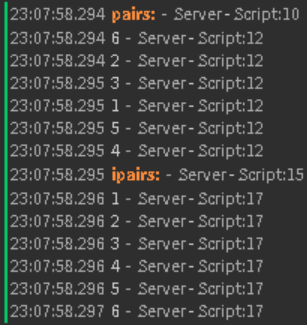# Ipairs vs pairs. What's the difference?

For the past 3 years whenever I do a for loop, I always see Ipairs next to pairs. Every time I google what it means, I don’t understand it, because they explain it very briefly without showing the use.
Can you explain to me the difference between pairs and Ipairs and then explain how I could use Ipairs. Thanks!

13 Likes

INHALE

9 Likes

But why would I ever choose ipairs instead of pairs?

I believe `pairs` indexes through the table (Dictionary preferably) in an unordered fashion, while `ipairs` goes through it in the order it was first made from (Or an Array)

`ipairs`/`pairs` is used depending on the order you want to sort your table from I believe (Not sure though, it’s something relevant along those lines)

(Oh yeah `ipairs` also stops if there is a `nil` value inside the table)

3 Likes
``````local numbers = {1,2,3,4,5}

for _,v in pairs(numbers) do

print(v)
end
``````

if you test that out with both ipairs and pairs, it’ll go in the same order, so that’s where I’m getting stuck.

1 Like

`ipairs` ensures that the indices are iterated in numerical order, e.g. 1, 2, 3, 4, 5 … #array. `ipairs` also ignores any key-value pairs (`{key = "value"}`)

`pairs` returns all keys and values out of order, although I’m pretty sure in Luau, if the array only consists of numerical indices that you don’t set by hand (like in the below example), then `pairs` would iterate the array in numerical order like `ipairs` does

``````local t = {
 = 6,
 = 4,
 = 3,
 = 1,
 = 2,
 = 5
}

warn("pairs:")
for i, v in pairs(t) do
print(v)
end

warn("ipairs:")
for i, v in ipairs(t) do
print(v)
end
``````You would use ipairs if your array’s numerical indices are out of order (for whatever reason).

52 Likes

Ohhhh…OHHHHHH OKAY! That makes sense now that I see the output, thanks for explaining it to me:) This will definitely help a bunch!

Hey there!

I may be wrong, but ipairs indexes with an integer, rather than indexing through the table. By this I mean, it loops through the table using the tables size (which can only be counted when the index of the entry in the table is an integer/number, as apposed to it being a string etc).

To simplify things:
`{ "Hi!", "How are you?" }` is the same thing as `{  = "Hi!",  = "How are you?" }`

Again, I may be wrong, but ipairs loops through the array using the integer/number in the brackets, since an integer in the index of a entry in a dictionary is the same as an entry in an array’s position. So it’s like it’s adding onto the integer its using to search the table until it reaches the table’s size. This ensures that it always loops chronologically in terms of each entry’s index in the table.

Note: Just saw a new explanation of this that’s a lot simpler, so go take a look at @heII_ish 's explanation for an easier to understand explanation!Hope this helps!2 Likes

Thanks for explaining, ipairs will help me a lot now:)!

Are there any situations where ipairs would be needed, instead of using pairs?

1 Like

I can think of one, something like a tournament. Think of it like this, you have a bunch of values and since the player values go in randomly and you need to find the biggest number you COULD do

local values = {}
local largest = 0

for _,v in pairs(values) do

if v < largest then

else
largest = v
end
end

and get the largest number that way, or you could simply use ipairs and do it that way.

2 Likes

I believe it’s just better practice to use `ipairs` on number-value pair arrays so numerical order is guaranteed. Also, `ipairs` stops iterating if it finds `nil` in the array

This would only print `1` because the second element is nil

``````for i, v in ipairs({ = 1,  = nil,  = 3}) do
print(v)
end
``````
2 Likes

`ipairs` basically only for number indexes. aka arrays, also it is faster than `pairs`.

1 Like

If you sorted your table using table.sort or a custom method, you wouldn’t be guaranteed to get that new order with pairs.

This could be to order a list, for example on a GUI. It could be for a podium at the end of a race. Literally anything where the order is critical, pairs cannot guarantee the order whatsoever.

In unsorted scenarios, e.g. a list of parts you want to perform an operation on and you don’t care which is first or last, then either is fine but ipairs will be faster.

1 Like
1 Like

for(int i = 0; i < n; i++){
//Code
}
LMAO

1 Like

lol

lua’s numeric for loop is pretty nice.

for start,end,step do
end

it’s just iterating thru tables that gets unintuitive with this random pairs/ipairs function. python’s generic for loop is nice. even c++ has a nice way of doing it now more modern way of looping through C++ arrays - Stack Overflow.

c#'s foreach method is great

1 Like